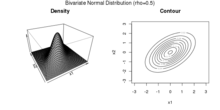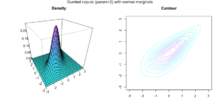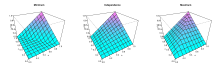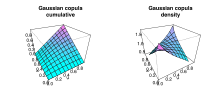# Copula (probability theory)

﻿
Copula (probability theory)

In probability theory and statistics, a copula can be used to describe the dependence between random variables. Copulas derive their name from linguistics.

The cumulative distribution function of a random vector can be written in terms of marginal distribution functions and a copula. The marginal distribution functions describe the marginal distribution of each component of the random vector and the copula describes the dependence structure between the components.

Copulas are popular in statistical applications as they allow one to easily model and estimate the distribution of random vectors by estimating marginals and copula separately. There are many parametric copula families available, which usually have parameters that control the strength of dependence. Some popular parametric copula models are outlined below.

## The basic idea

Consider a random vector$(X_1,X_2,\dots,X_d)$. Suppose its margins are continuous, i.e. the marginal CDFs$F_i(x) = \mathbb{P}[X_i\leq x]$ are continuous functions.

By applying probability integral transform to each component, the random vector$(U_1,U_2,\dots,U_d)=\left(F_1(X_1),F_2(X_2),\dots,F_d(X_d)\right)$

has uniform margins.

The copula of$(X_1,X_2,\dots,X_d)$ is defined as the joint cumulative distribution function of$(U_1,U_2,\dots,U_d)$:$C(u_1,u_2,\dots,u_d)=\mathbb{P}[U_1\leq u_1,U_2\leq u_2,\dots,U_d\leq u_d]$

The copula C contains all information on the dependence structure between the components of$(X_1,X_2,\dots,X_d)$ whereas the marginal cumulative distribution functions Fi contain all information on the marginal distributions.

Note that it is also possible to write$(X_1,X_2,\dots,X_d) = \left(F_1^{-1}(U_1),F_2^{-1}(U_2),\dots,F_d^{-1}(U_d)\right),$

which is used to simulate from$(X_1,X_2,\dots,X_d)$ in copula models. The inverses$F_i^{-1}$ are unproblematic as the Fi were assumed to be continuous. The analogous identity for the copula is$C(u_1,u_2,\dots,u_d)=\mathbb{P}[X_1\leq F_1^{-1}(u_1),X_2\leq F_2^{-1}(u_2),\dots,X_d\leq F_d^{-1}(u_d)]$

## Definition

In probabilistic terms,$C:[0,1]^d\rightarrow [0,1]$ is a d-dimensional copula if C is a joint cumulative distribution function of a d-dimensional random vector on the unit cube [0,1]d with uniform marginals.

In analytic terms,$C:[0,1]^d\rightarrow [0,1]$ is a d-dimensional copula if

•$C(u_1,\dots,u_{i-1},0,u_{i+1},\dots,u_d)=0$, the copula is zero if one of the arguments is zero,
•$C(1,\dots,1,u,1,\dots,1)=u$, the copula is equal to u if one argument is u and all others 1,
• C is hyperrectangle$B=\times_{i=1}^{d}[x_i,y_i]\subseteq [0,1]^d$ the C-volume of B is non-negative:$\int_B \mathrm{d}C(u) =\sum_{\mathbf z\in \times_{i=1}^{d}\{x_i,y_i\}} (-1)^{N(\mathbf z)} C(\mathbf z)\ge 0,$
where the$N(\mathbf z)=\#\{k : z_k=x_k\}$.

For instance, in the bivariate case,$C:[0,1]\times[0,1]\rightarrow [0,1]$ is a bivariate copula if C(0,u) = C(u,0) = 0, C(1,u) = C(u,1) = u and$C(y_1,y_2)-C(x_1,y_2)-C(y_1,x_2)+C(x_1,x_2) \geq 0$ for all$[x_1,y_1]\times[x_2,y_2]\subseteq [0,1]\times[0,1]$.

## Sklar's theoremDensity and contour plot of a Bivariate Gaussian DistributionDensity and contour plot of two Normal marginals joint with a Gumbel copula

Sklar's theorem provides the theoretical foundation for the application of copulas. Sklar's theorem states that a multivariate cumulative distribution function$H(x_1,\dots,x_d)=\mathbb{P}[X_1\leq x_1,\dots,X_d\leq x_d]$

of a random vector$(X_1,X_2,\dots,X_d)$ with margins$F_i(x) = \mathbb{P}[X_i\leq x]$ can be written as$H(x_1,\dots,x_d) = C\left(F_1(x_1),\dots,F_d(x_d) \right),$

where C is a copula.

The theorem also states that, given H, the copula is unique on$\operatorname{Ran}(F_1)\times\cdots\times \operatorname{Ran}(F_d)$, which is the cartesian product of the ranges of the marginal cdf's. This implies that the copula is unique if the margins Fi are continuous.

The converse is also true: given a copula$C:[0,1]^d\rightarrow [0,1]$ and margins Fi(x) then$C\left(F_1(x_1),\dots,F_d(x_d) \right)$ defines a d-dimensional cumulative distribution function.

## Fréchet–Hoeffding copula boundsGraphs of the bivariate Fréchet–Hoeffding copula limits and of the independence copula (in the middle).

The Fréchet–Hoeffding Theorem (after Maurice René Fréchet and Wassily Hoeffding ) states that for any Copula$C:[0,1]^d\rightarrow [0,1]$ and any$(u_1,\dots,u_d)\in[0,1]^d$ the following bounds hold:$W(u_1,\dots,u_d) \leq C(u_1,\dots,u_d) \leq M(u_1,\dots,u_d).$

The functions W is called lower Fréchet–Hoeffding bound and is defined as$W(u_1,\ldots,u_d) = \max\left\{1-d+\sum\limits_{i=1}^d {u_i} , 0 \right\}.$

The function M is called upper Fréchet–Hoeffding bound and is defined as$M(u_1,\ldots,u_d) = \min \{u_1,\dots,u_d\}.$

The upper bound is sharp: M is always a copula, it corresponds to comonotone random variables.

The lower bound is point-wise sharp, in the sense that for fixed u, there is a copula$\tilde{C}$ such that$\tilde{C}(u) = W(u)$. However, W is a copula only in two dimensions, in which case it corresponds to countermonotonic random variables.

In two dimensions, i.e. the bivariate case, the Fréchet–Hoeffding Theorem states$\max(u+v-1,0) \leq C(u,v) \leq \min\{u,v\}$

## Families of copulas

### Gaussian copulaCumulative and density distribution of Gaussian copula with ρ = 0.4

The Gaussian copula is constructed by projecting a multivariate normal distribution on$\mathbb{R}^d$ by means of the probability integral transform to the unit cube [0,1]d.

For a given correlation matrix$\Sigma\in\mathbb{R}^{d\times d}$, the Gaussian copula with parameter matrix Σ can be written as$C_\Sigma^{Gauss}(u) = \Phi_\Sigma\left(\Phi^{-1}(u_1),\dots, \Phi^{-1}(u_d) \right),$

where Φ − 1 is the inverse cumulative distribution function of a standard normal and ΦΣ is the joint cumulative distribution function of a multivariate normal distribution with mean vector zero and covariance matrix equal to the correlation matrix Σ.

The density can be written as$c_\Sigma^{Gauss}(u) = \frac{1}{\sqrt{\det{\Sigma}}}\exp\left(-\frac{1}{2} \begin{pmatrix}\Phi^{-1}(u_1)\\ \vdots \\ \Phi^{-1}(u_d)\end{pmatrix}^T \cdot \left(\Sigma^{-1}-\mathbf{I}\right) \cdot \begin{pmatrix}\Phi^{-1}(u_1)\\ \vdots \\ \Phi^{-1}(u_d)\end{pmatrix} \right),$

where$\mathbf{I}$ is the identity matrix.

### Archimedean copulas

Archimedean copulas are an associative class of copulas. Most common Archimedean copulas admit an explicit formula for the C, something not possible for instance for the Gaussian copula. In practise, Archimedean copulas are popular because they allow to model dependence in arbitrarily high dimensions with only one parameter, governing the strength of dependence.

A copula C is called Archimedean if it admits the representation$C(u_1,\dots,u_d) = \psi\left(\psi^{-1}(u_1)+\dots+\psi^{-1}(u_d)\right)\,$

where ψ is the so called generator.

The above formula yields a copula if and only if$\psi\,$ is d-monotone on$[0,\infty)$.  That is, if the kth derivatives of$\psi\,$ satisfy$(-1)^k\psi^{(k)}(x) \geq 0$

for all$x\geq 0$ and$k=0,1,\dots,d-2$ and ( − 1)d − 2ψd − 2(x) is nonincreasing and convex.

The generators in the following table are the most popular ones. All of them are completely monotone, i.e. d-monotone for all$d\in\mathbb{N}$.

Table with the most important generators
name generator$\,\psi(t)$ generator inverse$\,\psi^{-1}(t)$ parameter
Ali-Mikhail-Haq$\frac{1-\theta}{\exp(t)-\theta}$$\log\left(\frac{1-\theta+\theta t}{t}\right)$$\theta\in[0,1)$
Clayton$\left(1+t\right)^{-1/\theta}$$t^{-\theta}-1\,$$\theta\in(0,\infty)$
Frank$-\frac{\log(1-(1-\exp(-\theta))\exp(-t))}{\theta}$$-\log\left(\frac{\exp(-\theta t)-1}{\exp(-\theta)-1}\right)$$\theta\in(0,\infty)$
Gumbel$\exp\left(-t^{1/\theta}\right)$$\left(-\log(t)\right)^\theta$$\theta\in[1,\infty)$
Independence$\exp(-t)\,$$-\log(t)\,$
Joe$1-\left(1-\exp(-t)\right)^{1/\theta}$$-\log\left(1-(1-t)^\theta\right)$$\theta\in[1,\infty)$

## Empirical copulas

When studying multivariate data, one might want to investigate the underlying copula. Suppose have observations$(X_1^i,X_2^i,\dots,X_d^i), \, i=1,\dots,n$

from a random vector$(X_1,X_2,\dots,X_d)$ with continuous margins. The corresponding "true" copula observations would be$(U_1^i,U_2^i,\dots,U_d^i)=\left(F_1(X_1^i),F_2(X_2^i),\dots,F_d(X_d^i)\right), \, i=1,\dots,n.$

However, the marginal distribution functions Fi are usually not known. Therefore, one can construct pseudo copula observations by using the empirical distribution functions$F_k^n(x)=\frac{1}{n} \sum_{i=1}^n \mathbf{1}(X_k^i\leq x)$

instead. Then, the pseudo copula observations are defined as$(\tilde{U}_1^i,\tilde{U}_2^i,\dots,\tilde{U}_d^i)=\left(F_1^n(X_1^i),F_2^n(X_2^i),\dots,F_d^n(X_d^i)\right), \, i=1,\dots,n.$

The corresponding empirical copula is then defined as$C^n(u_1,\dots,u_d) = \frac{1}{n} \sum_{i=1}^n \mathbf{1}\left(\tilde{U}_1^i\leq u_1,\dots,\tilde{U}_d^i\leq u_d\right).$

The components of the pseudo copula samples can also be written as$\tilde{U}_k^i=R_k^i/n$, where$R_k^i$ is the rank of the observation$X_k^i$:$R_k^i=\sum_{j=1}^n \mathbf{1}(X_k^j\leq X_k^i)$

Therefore, the empirical copula can be seen as the empirical distribution of the rank transformed data.

## Monte Carlo integration for copula models

In statistical applications, many problems can be formulated in the following way. One is interested in the expectation of a response function$g:\mathbb{R}^d\rightarrow\mathbb{R}$ applied to some random vector$(X_1,\dots,X_d)$. If we denote the cdf of this random vector with H, the quantity of interest can thus be written as$\mathbb{E}\left[g(X_1,\dots,X_d)\right]=\int_{\mathbb{R}^d}g(x_1,\dots,x_d)\mathrm{d}H(x_1,\dots,x_d).$

If H is given by a copula model, i.e.,$H(x_1,\dots,x_d)=C(F_1(x_1),\dots,F_d(x_d))$

this expectation can be rewritten as$\mathbb{E}\left[g(X_1,\dots,X_d)\right]=\int_{[0,1]^d}g(F_1^{-1}(u_1),\dots,F_d^{-1}(u_d))\mathrm{d}C(u_1,\dots,u_d).$

In case the copula C is absolutely continuous, i.e. C has a density c, this equation can be written as$\mathbb{E}\left[g(X_1,\dots,X_d)\right]=\int_{[0,1]^d}g(F_1^{-1}(u_1),\dots,F_d^{-1}(u_d))c(u_1,\dots,u_d)\mathrm{d}u_1\cdots\mathrm{d}u_d.$

If copula and margins are known (or if they have been estimated), this expectation can be approximated through the following Monte Carlo algorithm:

1. Draw a sample$(U_1^k,\dots,U_d^k)\sim C\;\;(k=1,\dots,n)$ of size n from the copula C
2. By applying the inverse marginal cdf's, produce a sample of$(X_1,\dots,X_d)$ by setting$(X_1^k,\dots,X_d^k)=(F_1^{-1}(U_1^k),\dots,F_d^{-1}(U_d^k))\sim H\;\;(k=1,\dots,n)$
3. Approximate$\mathbb{E}\left[g(X_1,\dots,X_d)\right]$ by its empirical value:$\mathbb{E}\left[g(X_1,\dots,X_d)\right]\approx \frac{1}{n}\sum_{k=1}^n g(X_1^k,\dots,X_d^k)$

## Applications

### Quantitative finance

The applications of copulas in quantitative finance are numerous, both in the real-world probability of risk/portfolio management and in the risk-neutral probability of derivatives pricing.

In risk/portfolio management, copulas are used to perform stress-tests and robustness checks: panic copulas are glued with market estimates of the marginal distributions to analyze the effects of panic regimes on the portfolio profit and loss distribution. Panic copulas are created by Monte Carlo simulation, mixed with a re-weighting of the probability of each scenario.

As far as derivatives pricing is concerned, dependence modelling with copula functions is widely used in applications of financial risk assessment and actuarial analysis – for example in the pricing of collateralized debt obligations (CDOs). Some believe the methodology of applying the Gaussian copula to credit derivatives to be one of the reasons behind the global financial crisis of 2008–2009. Despite this perception, there are documented attempts of the financial industry, occurring before the crisis, to address the limitations of the Gaussian copula and of copula functions more generally, specifically the lack of dependence dynamics[clarification needed] and the poor representation of extreme events. There have been attempts to propose models rectifying some of the copula limitations.

While the application of copulas in credit has gone through popularity as well as misfortune during the global financial crisis of 2008–2009, it is arguably an industry standard model for pricing CDOs. Less arguably, copulas have also been applied to other asset classes as a flexible tool in analyzing multi-asset derivative products. The first such application outside credit was to use a copula to construct an implied basket volatility surface, taking into account the volatility smile of basket components. Copulas have since gained popularity in pricing and risk management  of options on multi-assets in the presence of volatility smile/skew, in equity, foreign exchange and fixed income derivative business. Some typical example applications of copulas are listed below:

• Analyzing and pricing volatility smile/skew of exotic baskets, e.g. best/worst of;
• Analyzing and pricing volatility smile/skew of less liquid FX[clarification needed] cross, which is effectively a basket: C = S1/S2 or C = S1*S2;
• Analyzing and pricing spread options, in particular in fixed income constant maturity swap spread options.

### Civil engineering

Recently, copula functions have been successfully applied to the database formulation for the reliability analysis of highway bridges, and to various multivariate simulation studies in civil, mechanical and offshore engineering.[citation needed]

### Medicine

Copula functions have been successfully applied to the analysis of spike counts in neuroscience 

### Weather research

Copulas have been extensively used in climate and weather related research.

Wikimedia Foundation. 2010.

### Look at other dictionaries:

• Independence (probability theory) — In probability theory, to say that two events are independent intuitively means that the occurrence of one event makes it neither more nor less probable that the other occurs. For example: The event of getting a 6 the first time a die is rolled… …   Wikipedia

• Copula — Not to be confused with cupola, an architectural term with similar spelling. Copula may refer to: copula (linguistics), a word used to link subject and predicate Indo European copula, this word in the Indo European languages copula (music), a… …   Wikipedia

• Probability distribution — This article is about probability distribution. For generalized functions in mathematical analysis, see Distribution (mathematics). For other uses, see Distribution (disambiguation). In probability theory, a probability mass, probability density …   Wikipedia

• List of probability topics — This is a list of probability topics, by Wikipedia page. It overlaps with the (alphabetical) list of statistical topics. There are also the list of probabilists and list of statisticians.General aspects*Probability *Randomness, Pseudorandomness,… …   Wikipedia

• List of probability distributions — Many probability distributions are so important in theory or applications that they have been given specific names.Discrete distributionsWith finite support* The Bernoulli distribution, which takes value 1 with probability p and value 0 with… …   Wikipedia

• Joint probability distribution — In the study of probability, given two random variables X and Y that are defined on the same probability space, the joint distribution for X and Y defines the probability of events defined in terms of both X and Y. In the case of only two random… …   Wikipedia

• Wikipedia:Artículos solicitados — Atajos WP:AS WP:SOL Artículos solicitados En esta página pue …   Wikipedia Español

• Wikiproyecto:Matemáticas — …   Wikipedia Español

• List of statistics topics — Please add any Wikipedia articles related to statistics that are not already on this list.The Related changes link in the margin of this page (below search) leads to a list of the most recent changes to the articles listed below. To see the most… …   Wikipedia

• logic, history of — Introduction       the history of the discipline from its origins among the ancient Greeks to the present time. Origins of logic in the West Precursors of ancient logic       There was a medieval tradition according to which the Greek philosopher …   Universalium